ENGR338 Lab 2022 Fall
Lab 6
More gates

NAND, NOR , XOR, AND FULL ADDER

Introduction: This lab uses Electric VLSI and LTSpice to simulate pmos and nmos gates in order to create an inverter.

Materials: LTSpice, Electric VLSI

Methods: First, an NAND gate was created using 2 PMOS gates in parallel and 2 NMOS gates in series as seen in Figure 1 below. I am just now realizing that I forgot to put the circle on the NAND gate so that was a mistake but otherwise the gate functioned.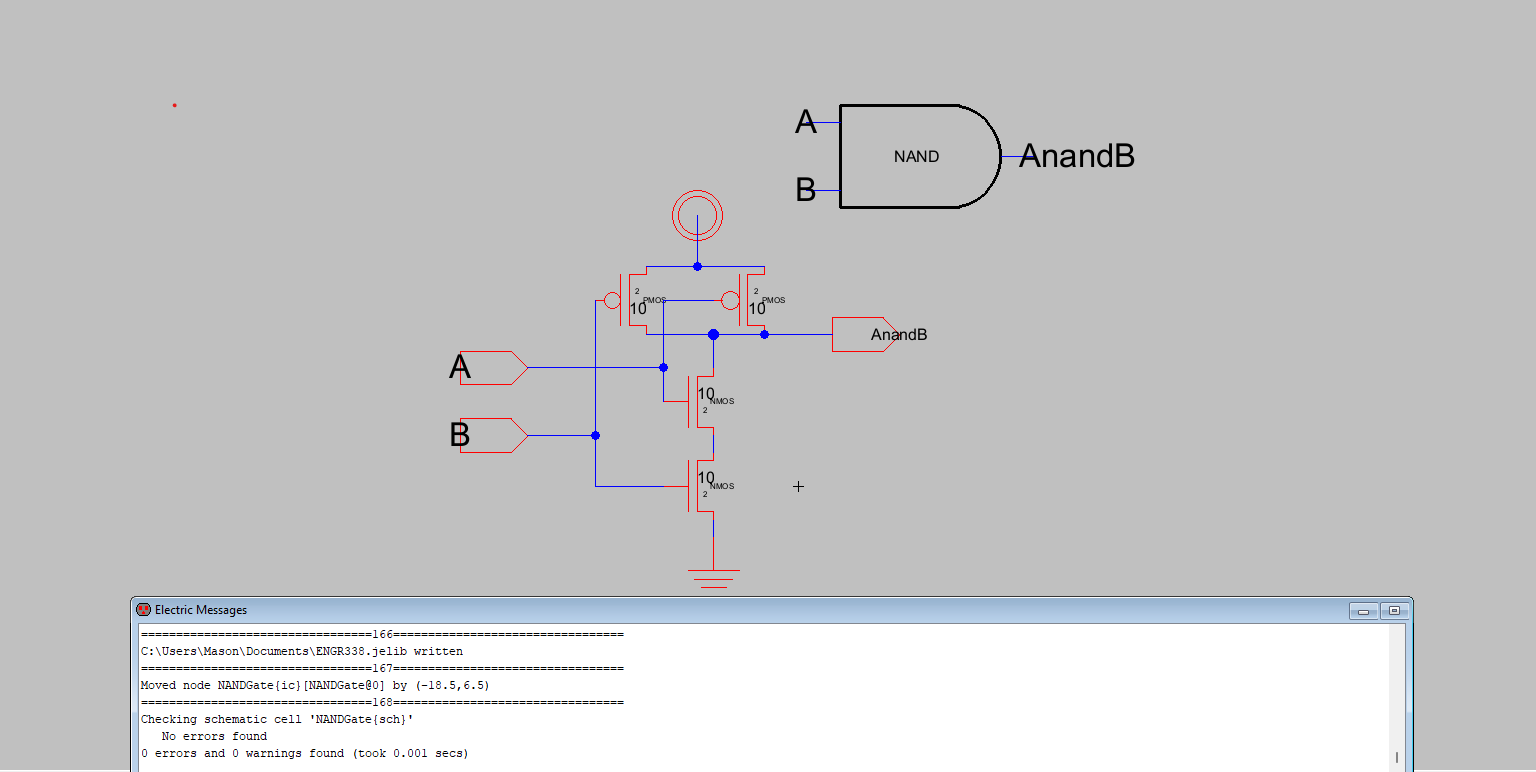Figure 1. NAND Schematic and Icon with 0 DRC Errors.

The circuit was then simulated in LTSpice as seen in Figure 2.Figure 2. NAND Simulation.

The NAND gate layout was then completed fairly easily and passed the NCC check and DRC with 0 errors which can be seen in figure 3.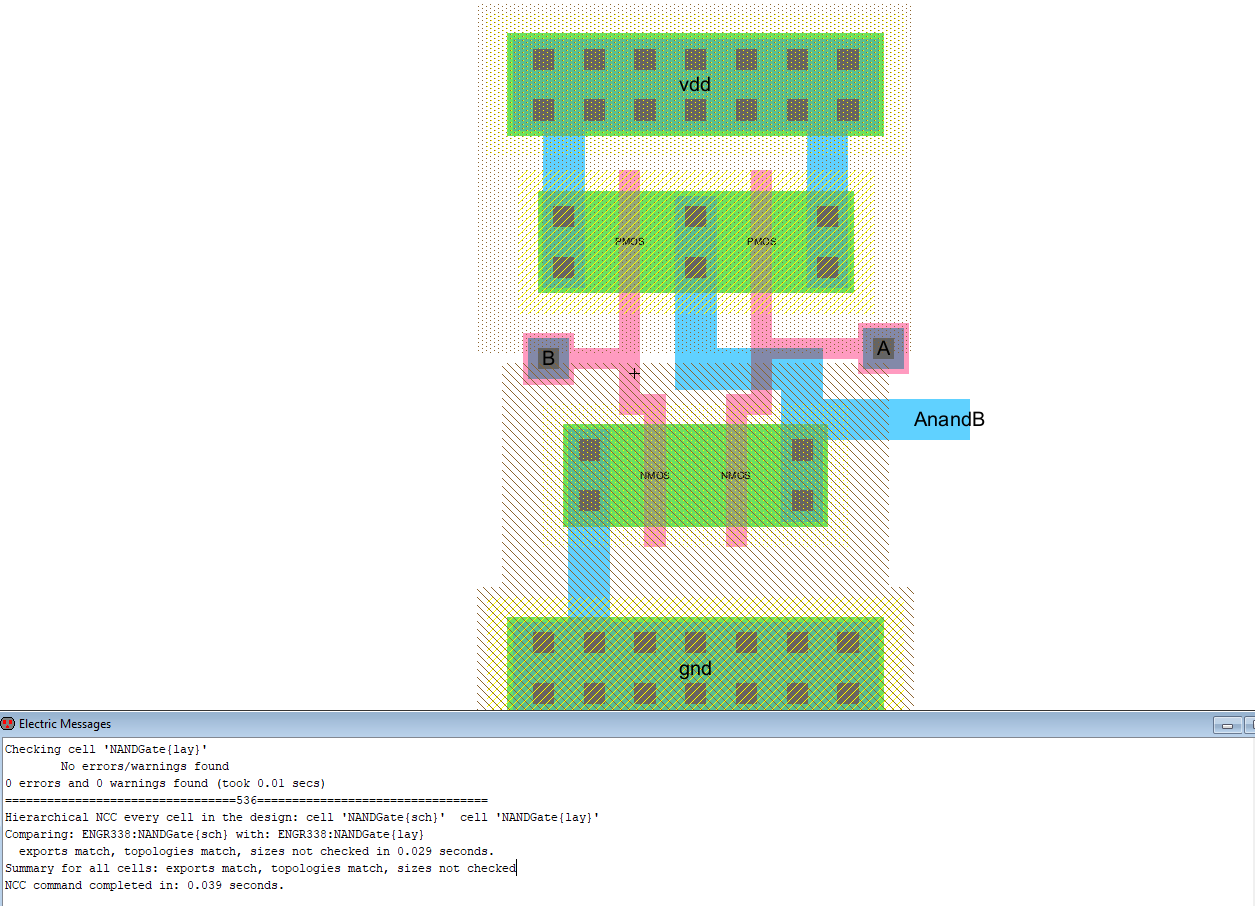Figure 3. NAND Gate layout.

The same process was then used for the NOR gate. The schematic can be seen below in Figure 4. The Layout can be seen in Figure 5.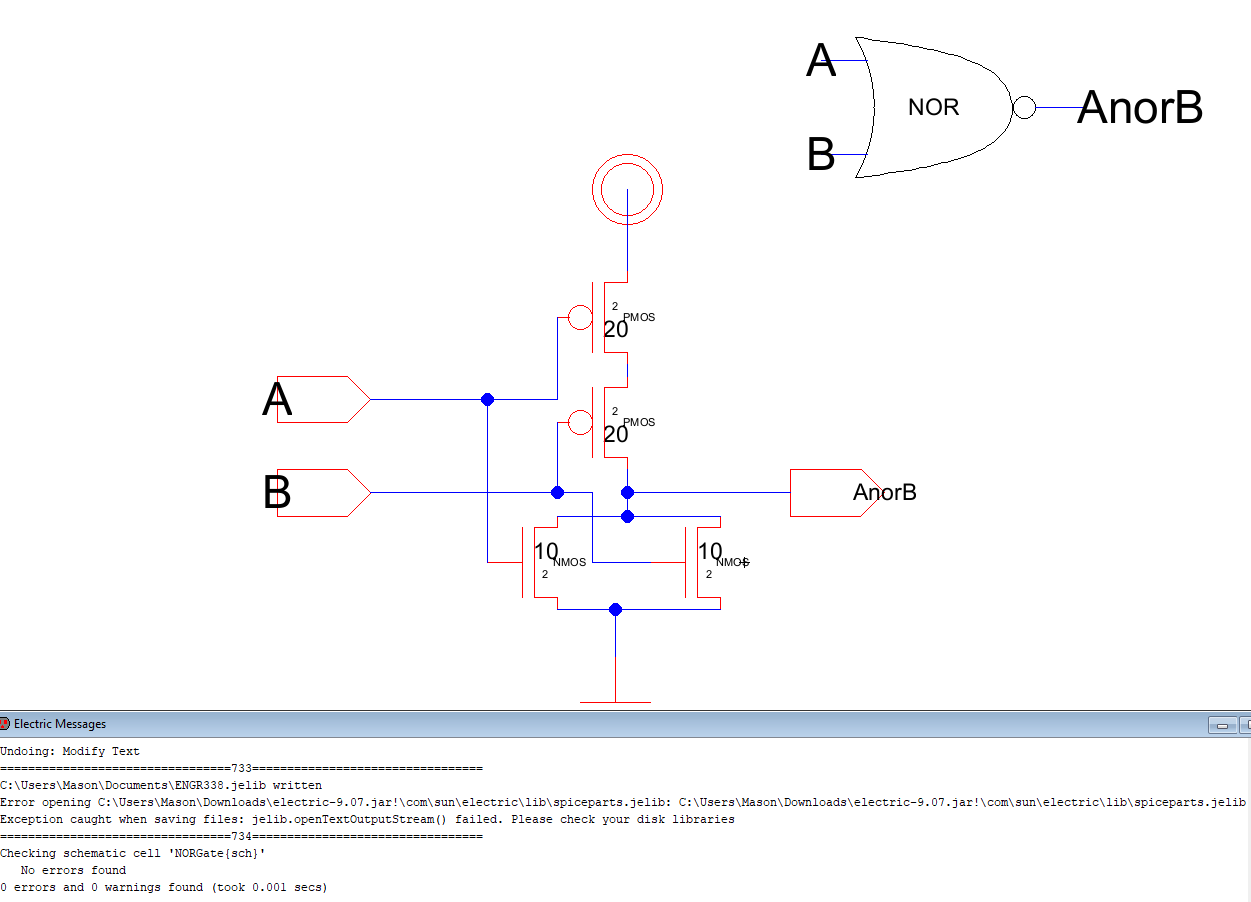Figure 4. NOR Gate schematic and icon.

This process went fairly smoothly with no issues.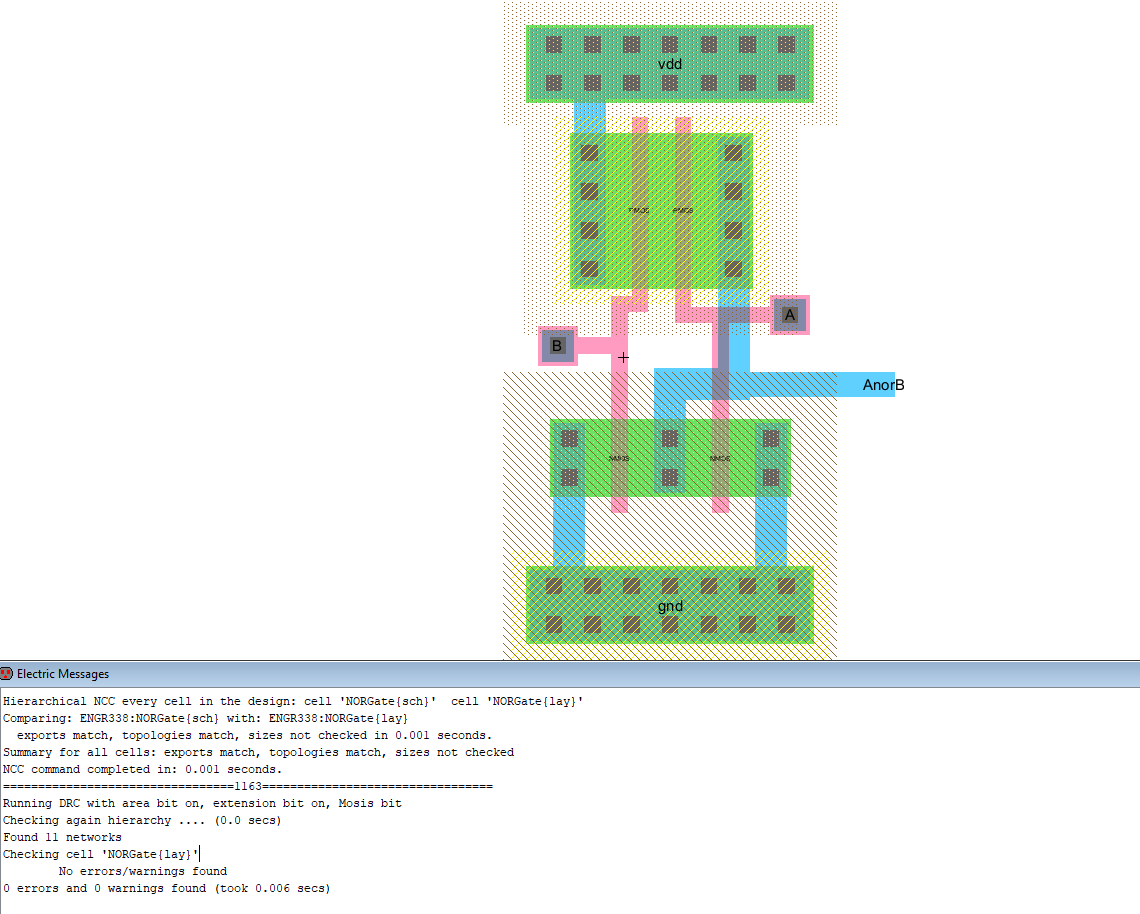Figure 5. NOR Gate Layout.

The final gate needed for the Full Adder was an Xor gate. This gate was layed out using NMOS and PMOS gates. Two inverters were created since both A and Anot as well as B and Bnot are needed for the Xor gate. The schematic for the Xor gate can be seen below in Figure 6.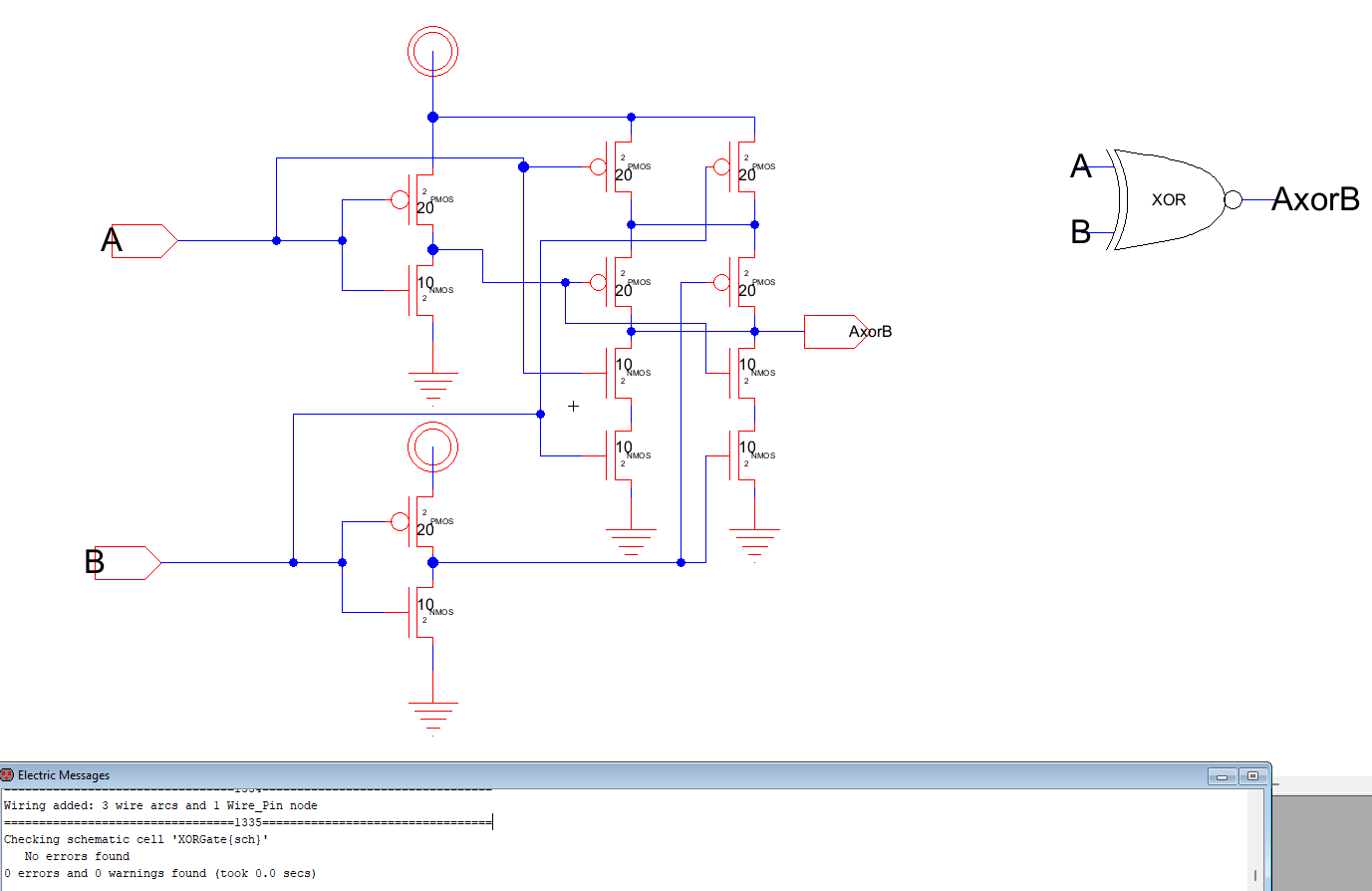Figure 6. Xor gate layout with clean DRC check.

A stick diagram was used to layout the Xor gate layout before going into electric. I tried to use different colors but it ended up confusing me more.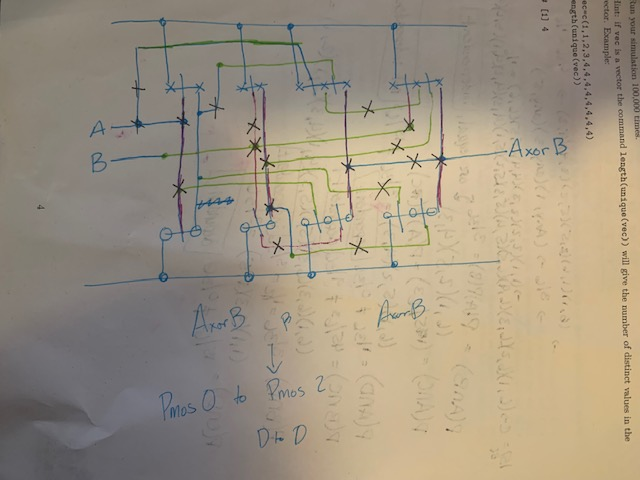Figure 7. Stick diagram for Xor Gate(on a stats exam, I ran out of paper).

The schematic was then layed out... several times... way too many times to be proud of it but I finally finished the layout and it can be seen below in Figure 8.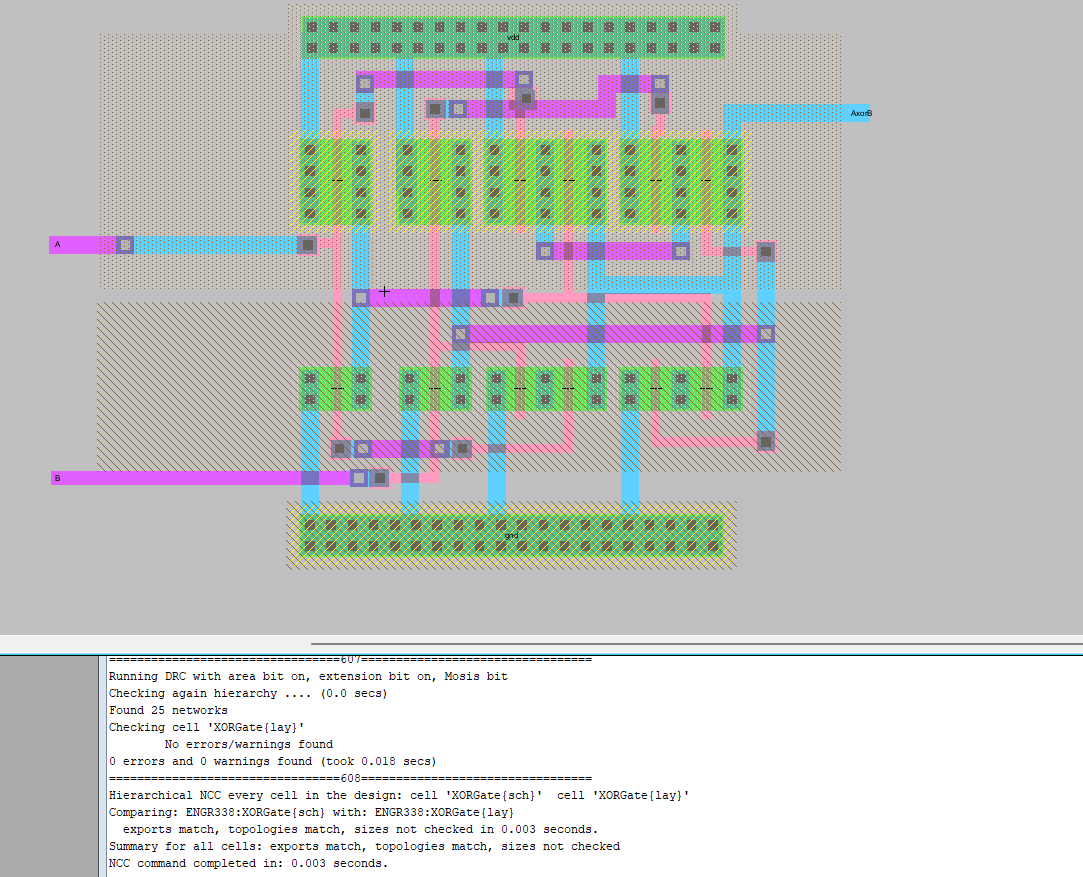Figure 8. XOR Gate Layout with Clean NCC and DRC!

The final step of this lab was to make the full adder. This was a lot easier than I thought it would be. The schematic was layed out as seen below in Figure 9. The logic was found on a tutorial posted to yilectronics.com.The final and most daunting task of the lab was the layout for the full adder. This was also a lot easier than I expected since all of the gates were known to work they just had to be wired together the final layout can be seen in Figure 10.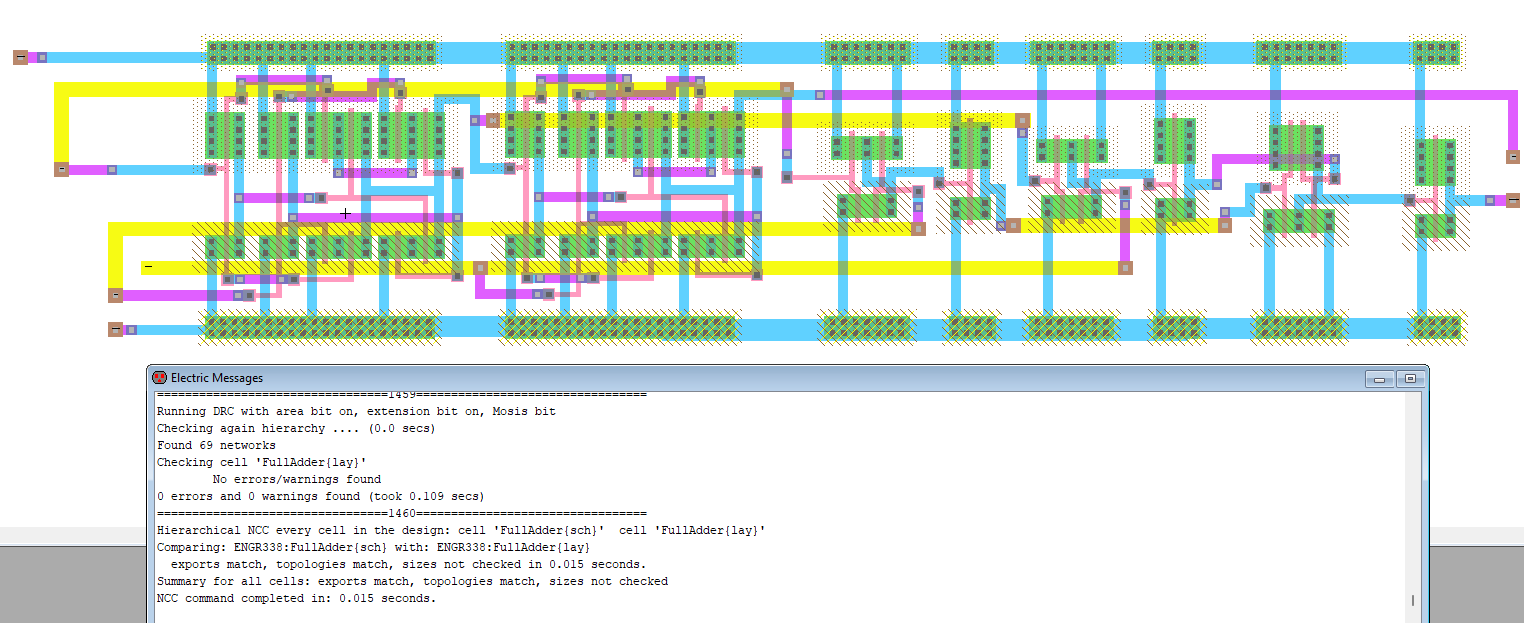All of the aformentioned circuits except the Full adder were simulated driving a 100f capacitors to ensure the logic was correct and can be seen in the results section. The full adder was also simulated below in the results section.

Results:

As mentioned above the NAND, NOR, and XOR gates were simulated driving a 100fF capacitor as seen below in Figures 11-13.Figure 11. Simulation
results for the NAND gate wired to a 100fF capacitor.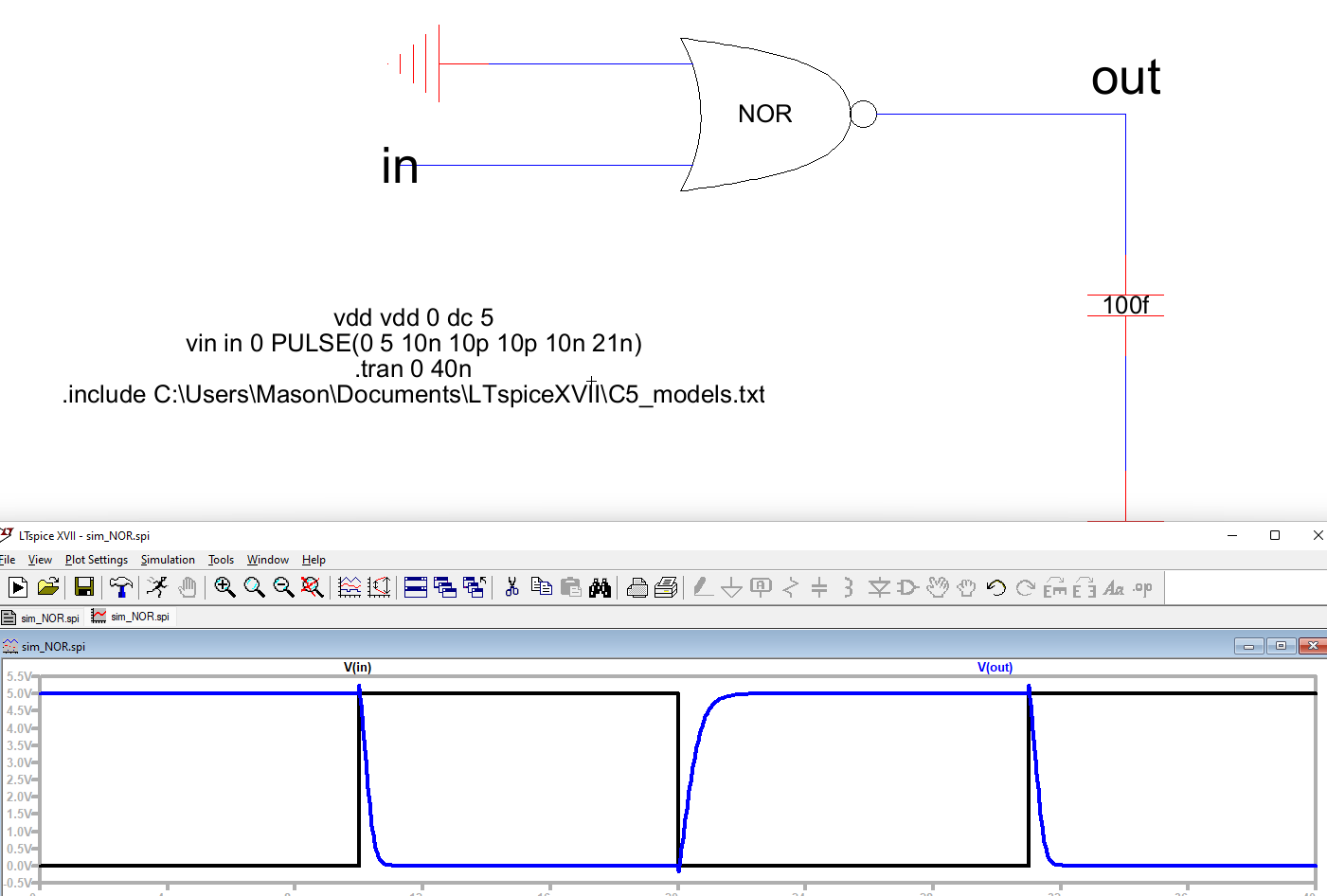Figure 12. Simulation results for the NOR gate wired to a 100fF capacitor and ground.

And finally the XOR gate.Figure 13. Simulation results for the XOR gate wired to a 100fF capacitor and VDD.

The XOR gate was then wired to VDD to ensure the logic was working.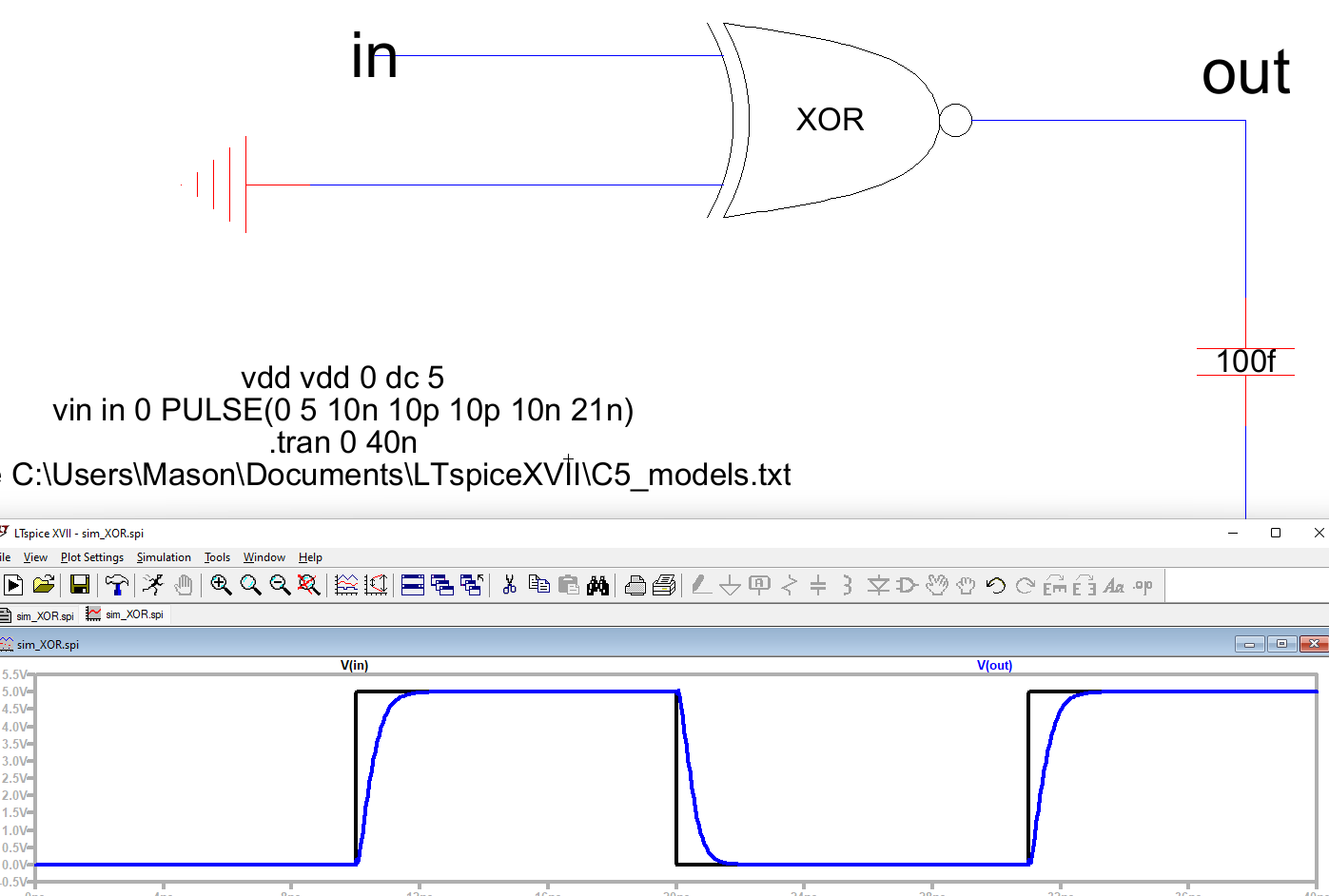Figure 14. Simulation results for the XOR gate wired to a 100fF capacitor and GND.

Finally, the full adders logic was simulatedFigure 15. Simulation results for the Full adder with Cin shorted to ground.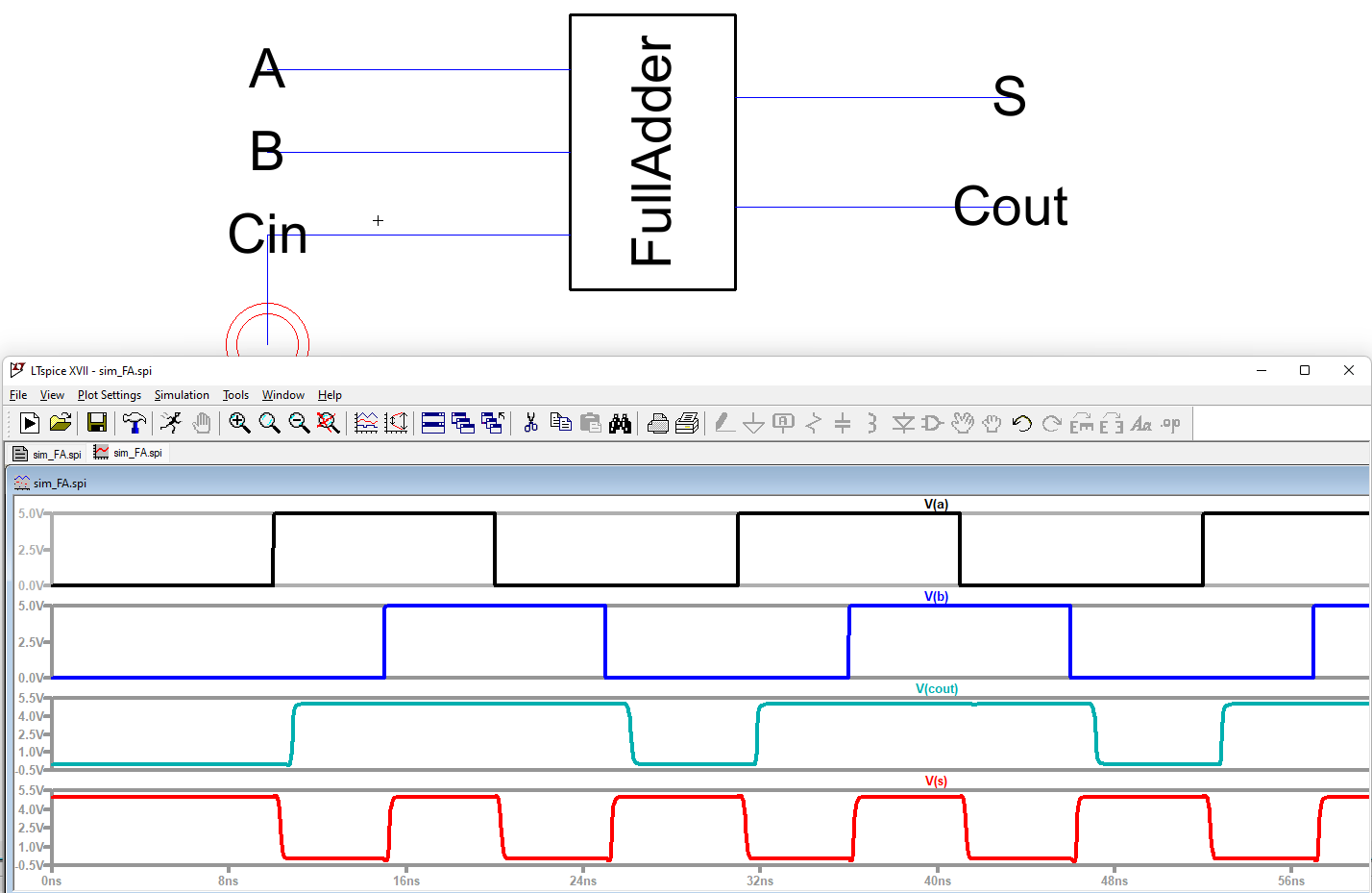Figure 16. Simulation results for the Full adder with Cin shorted to VDD.

Discussion: Holy cow did these layouts get complicated really fast. I had a lot of errors with Electric not wanting to boot so I had to wipe my settings a few times in the registry. I also had to do this in SEVERAL sittings as to not get too frustrated. At the end of the day none of it was too technically complex it was just very easy to miss things and the debugger for NCC wasn't always the most useful.